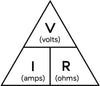# Ohm's Law Calculator

Ohm's law states that the current passing through a conductor between two points is directly proportional to the voltage across the two points, and inversely proportional to the resistance between them. This relationship is described by the following equation:

I = V / R or displayed as:This used by crossing out the value to which you want the result. Where I is the current in amperes (A), V is the voltage in volts (V), and R is the resistance ohms (Ω). This how I remember the formula.

## Generate results with our custom calculator

Enter the values below to calculate the unknown value:

Voltage (V):
Current (I):
Resistance (R):

Voltage Current Resistance